## Example 38.1 Generalized Additive Model with Binary Data

This example illustrates the capabilities of the GAM procedure and compares it to the GENMOD procedure.From this example, you can see that PROC GAM is very useful in visualizing the data and detecting the nonlinearity among the variables.

The data used in this example are based on a study by Bell et al. (1994). Bell and his associates studied the result of multiple-level thoracic and lumbar laminectomy, a corrective spinal surgery commonly performed on children. The data in the study consist of retrospective measurements on 83 patients. The specific outcome of interest is the presence (1) or absence (0) of kyphosis, defined as a forward flexion of the spine of at least 40 degrees from vertical. The available predictor variables are age in months at time of the operation (Age), the starting of vertebrae levels involved in the operation (StartVert), and the number of levels involved (NumVert). The goal of this analysis is to identify risk factors for kyphosis. PROC GENMOD can be used to investigate the relationship among kyphosis and the predictors. The following statements create the data kyphosis and fit a logistic model specifying linear effects for the three predictors:

```title 'Comparing PROC GAM with PROC GENMOD';
data kyphosis;
input Age StartVert NumVert Kyphosis @@;
datalines;
71  5  3  0     158 14 3 0    128 5   4 1
2   1  5  0     1   15 4 0    1   16  2 0
61  17 2  0     37  16 3 0    113 16  2 0
59  12 6  1     82  14 5 1    148 16  3 0
18  2  5  0     1   12 4 0    243 8   8 0
168 18 3  0     1   16 3 0    78  15  6 0
175 13 5  0     80  16 5 0    27  9   4 0
22  16 2  0     105 5  6 1    96  12  3 1
131 3  2  0     15  2  7 1    9   13  5 0
12  2 14  1     8   6  3 0    100 14  3 0
4   16 3  0     151 16 2 0    31  16  3 0
125 11 2  0     130 13 5 0    112 16  3 0
140 11 5  0     93  16 3 0    1   9   3 0
52  6  5  1     20  9  6 0    91  12  5 1
73  1  5  1     35  13 3 0    143 3   9 0
61  1  4  0     97  16 3 0    139 10  3 1
136 15 4  0     131 13 5 0    121 3   3 1
177 14 2  0     68  10 5 0    9   17  2 0
139 6  10 1     2   17 2 0    140 15  4 0
72  15 5  0     2   13 3 0    120 8   5 1
51  9  7  0     102 13 3 0    130 1   4 1
114 8  7  1     81  1  4 0    118 16  3 0
118 16 4  0     17  10 4 0    195 17  2 0
159 13 4  0     18  11 4 0    15  16  5 0
158 15 4  0     127 12 4 0    87  16  4 0
206 10 4  0     11  15 3 0    178 15  4 0
157 13 3  1     26  13 7 0    120 13  2 0
42  6  7  1     36  13 4 0
;
```
```proc genmod data=kyphosis descending;
model Kyphosis = Age StartVert NumVert/link=logit dist=binomial;
run;
```

The GENMOD analysis of the independent variable effects is shown in Output 38.1.1. Based on these results, the only significant factor is StartVert with a log odds ratio of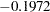. The variable NumVert has a p-value of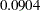with a log odds ratio of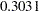.

Output 38.1.1 GENMOD Analysis: Partial Output
 Comparing PROC GAM with PROC GENMOD

The GENMOD Procedure

Analysis Of Maximum Likelihood Parameter Estimates
Parameter DF Estimate Standard Error Wald 95% Confidence Limits Wald Chi-Square Pr > ChiSq
Intercept 1 -1.2497 1.2424 -3.6848 1.1853 1.01 0.3145
Age 1 0.0061 0.0055 -0.0048 0.0170 1.21 0.2713
StartVert 1 -0.1972 0.0657 -0.3260 -0.0684 9.01 0.0027
NumVert 1 0.3031 0.1790 -0.0477 0.6540 2.87 0.0904
Scale 0 1.0000 0.0000 1.0000 1.0000

 Note: The scale parameter was held fixed.

The GENMOD procedure assumes a strict linear relationship between the predictors and the response function, which is the logit (log odds) in this model. The following SAS statements use PROC GAM to investigate a less restrictive model, with moderately flexible spline terms for each of the predictors:

```title 'Comparing PROC GAM with PROC GENMOD';
proc gam data=kyphosis;
model Kyphosis (event='1') = spline(Age      ,df=3)
spline(StartVert,df=3)
spline(NumVert  ,df=3) / dist=binomial;
run;
```

The MODEL statement requests an additive model with a univariate smoothing spline for each term. The response variable option EVENT= chooses Kyphosis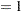(presence) as the event so that the probability of presence of kyphosis is modeled. The option “DIST=BINOMIAL” with binary responses specifies a logistic model. Each term is fit by using a univariate smoothing spline with three degrees of freedom. Of these three degrees of freedom, one is taken up by the linear portion of the fit and two are left for the nonlinear spline portion. Although this might seem to be an unduly modest amount of flexibility, it is better to be conservative with a data set this small.

Output 38.1.2 and Output 38.1.3 list the output from PROC GAM.

Output 38.1.2 Summary Statistics
 Comparing PROC GAM with PROC GENMOD

The GAM Procedure
Dependent Variable: Kyphosis
Smoothing Model Component(s): spline(Age) spline(StartVert) spline(NumVert)

Summary of Input Data Set
Number of Observations 83
Number of Missing Observations 0
Distribution Binomial

Response Profile
Ordered
Value
Kyphosis Total
Frequency
1 0 65
2 1 18

 Note: PROC GAM is modeling the probability that Kyphosis=1. One way to change this to model the probability that Kyphosis=0 is to specify the response variable option EVENT='0'.

Iteration Summary and Fit Statistics
Number of local scoring iterations 9
Local scoring convergence criterion 2.6635661E-9
Final Number of Backfitting Iterations 1
Final Backfitting Criterion 5.2326593E-9
The Deviance of the Final Estimate 46.610922438

Output 38.1.3 Model Fit Statistics
Regression Model Analysis
Parameter Estimates
Parameter Parameter
Estimate
Standard
Error
t Value Pr > |t|
Intercept -2.01533 1.45620 -1.38 0.1706
Linear(Age) 0.01213 0.00794 1.53 0.1308
Linear(StartVert) -0.18615 0.07628 -2.44 0.0171
Linear(NumVert) 0.38347 0.19102 2.01 0.0484

Smoothing Model Analysis
Fit Summary for Smoothing Components
Component Smoothing
Parameter
DF GCV Num
Unique
Obs
Spline(Age) 0.999996 2.000000 328.512831 66
Spline(StartVert) 0.999551 2.000000 317.646685 16
Spline(NumVert) 0.921758 2.000000 20.144056 10

Smoothing Model Analysis
Analysis of Deviance
Source DF Sum of Squares Chi-Square Pr > ChiSq
Spline(Age) 2.00000 10.494369 10.4944 0.0053
Spline(StartVert) 2.00000 5.494968 5.4950 0.0641
Spline(NumVert) 2.00000 2.184518 2.1845 0.3355

The critical part of the GAM results is the "Analysis of Deviance" table, shown in Output 38.1.3. For each smoothing effect in the model, this table gives a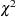test comparing the deviance between the full model and the model without the nonparametric component of this variable. The analysis of deviance results indicate that the nonparametric effect of Age is highly significant, the nonparametric effect of StartVert is nearly significant, and the nonparametric effect of NumVert is insignificant at the 5% level.

PROC GAM can also perform approximate analysis of deviance for smoothing effects by using the ANODEV=NOREFIT option, as in the following statements:

```title 'PROC GAM with Approximate Analysis of Deviance';
proc gam data=kyphosis;
model Kyphosis (event='1') = spline(Age      ,df=3)
spline(StartVert,df=3)
spline(NumVert  ,df=3) /
dist=binomial anodev=norefit;
run;
```

Output 38.1.4 Approximate Analysis of Deviance Table
 PROC GAM with Approximate Analysis of Deviance

The GAM Procedure
Dependent Variable: Kyphosis
Smoothing Model Component(s): spline(Age) spline(StartVert) spline(NumVert)

Smoothing Model Analysis
Approximate Analysis of Deviance
Source DF Chi-Square Pr > ChiSq
Spline(Age) 2.00000 7.0888 0.0289
Spline(StartVert) 2.00000 5.0431 0.0803
Spline(NumVert) 2.00000 2.2471 0.3251

The "Approximate Analysis of Deviance" table shown in Output 38.1.4 yields similar conclusions to those of the "Analysis of Deviance" table (Output 38.1.3). In addition to fitting the model using all the specified smoothing effects, the default ANODEV=REFIT option requires fittingadditional subset models tosmoothing effects. Each submodel is fit by omitting one smoothing term from the model. By contrast, the ANODEV=NOREFIT option keeps the nonparametric terms fixed and requires a weighted least squares fit for only the parametric part of the model. Hence, GAM with the ANODEV=NOREFIT option is computationally inexpensive and is useful for obtaining approximate analysis of deviance results for models with many smoothing effects. This option assumes that the remaining nonparametric terms do not change much with the deletion of one nonparametric component. It should be used with caution when a model contains highly correlated predictors.

Plots of the partial predictions for each predictor can be used to investigate why PROC GAM and PROC GENMOD produce different results. The following statements use ODS Graphics to produce plots of the individual smoothing components. The CLM suboption in the PLOTS=COMPONENTS option adds a curvewise Bayesian confidence band to each smoothing component, while the COMMONAXES suboption forces all three smoothing component plots to share the same vertical axis limits, allowing a visual judgment of the relative nonparametric effect sizes.

```ods graphics on;

proc gam data=kyphosis plots=components(clm commonaxes);
model Kyphosis (event='1') = spline(Age      ,df=3)
spline(StartVert,df=3)
spline(NumVert  ,df=3) / dist=binomial;
run;

ods graphics off;
```

For general information about ODS Graphics, see Chapter 21, Statistical Graphics Using ODS. For specific information about the graphics available in the GAM procedure, see the section ODS Graphics. The smoothing component plots are displayed in Output 38.1.5.

Output 38.1.5 Partial Prediction for Each Predictor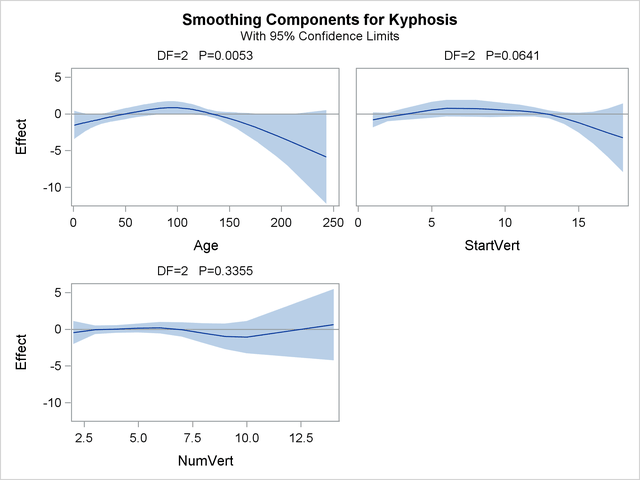The plots show that the partial predictions corresponding to both Age and StartVert have a quadratic pattern, while NumVert has a more complicated but ultimately nonsignificant pattern.

An important difference between the first analysis of these data with GENMOD and the subsequent analysis with GAM is that GAM indicates that Age has a significant but nonlinear association with kyphosis. The difference is due to the fact that the GENMOD model includes only the linear effect of Age whereas the GAM model allows a more complex relationship, which the plots indicate is nearly quadratic. Having used the GAM procedure to discover an appropriate form of the dependence of Kyphosis on each of the three independent variables, you can use the GENMOD procedure to fit and assess the corresponding parametric model. The following statements fit a GENMOD model with quadratic terms for all three variables. The parameter estimates are shown in Output 38.1.6.

```title 'Comparing PROC GAM with PROC GENMOD';
proc genmod data=kyphosis descending;
model kyphosis = Age       Age      *Age
StartVert StartVert*StartVert
NumVert   NumVert  *NumVert /
run;
```

Output 38.1.6 Logistic Model with Quadratic Terms
 Comparing PROC GAM with PROC GENMOD

The GENMOD Procedure

Analysis Of Maximum Likelihood Parameter Estimates
Parameter DF Estimate Standard Error Wald 95% Confidence Limits Wald Chi-Square Pr > ChiSq
Intercept 1 -5.8134 2.5618 -10.8345 -0.7923 5.15 0.0233
Age 1 0.0819 0.0345 0.0143 0.1496 5.63 0.0176
Age*Age 1 -0.0004 0.0002 -0.0008 -0.0000 4.32 0.0376
StartVert 1 0.4394 0.3234 -0.1944 1.0733 1.85 0.1742
StartVert*StartVert 1 -0.0396 0.0202 -0.0791 -0.0001 3.86 0.0495
NumVert 1 0.3798 0.5988 -0.7939 1.5535 0.40 0.5259
NumVert*NumVert 1 0.0020 0.0420 -0.0803 0.0843 0.00 0.9621
Scale 0 1.0000 0.0000 1.0000 1.0000

 Note: The scale parameter was held fixed.

The p-value for thetest is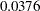for dropping the quadratic term of Age,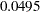for dropping the quadratic term of StartVert, andfor dropping this quadratic term of NumVert. The results for the quadratic GENMOD model are consistent with the GAM results.